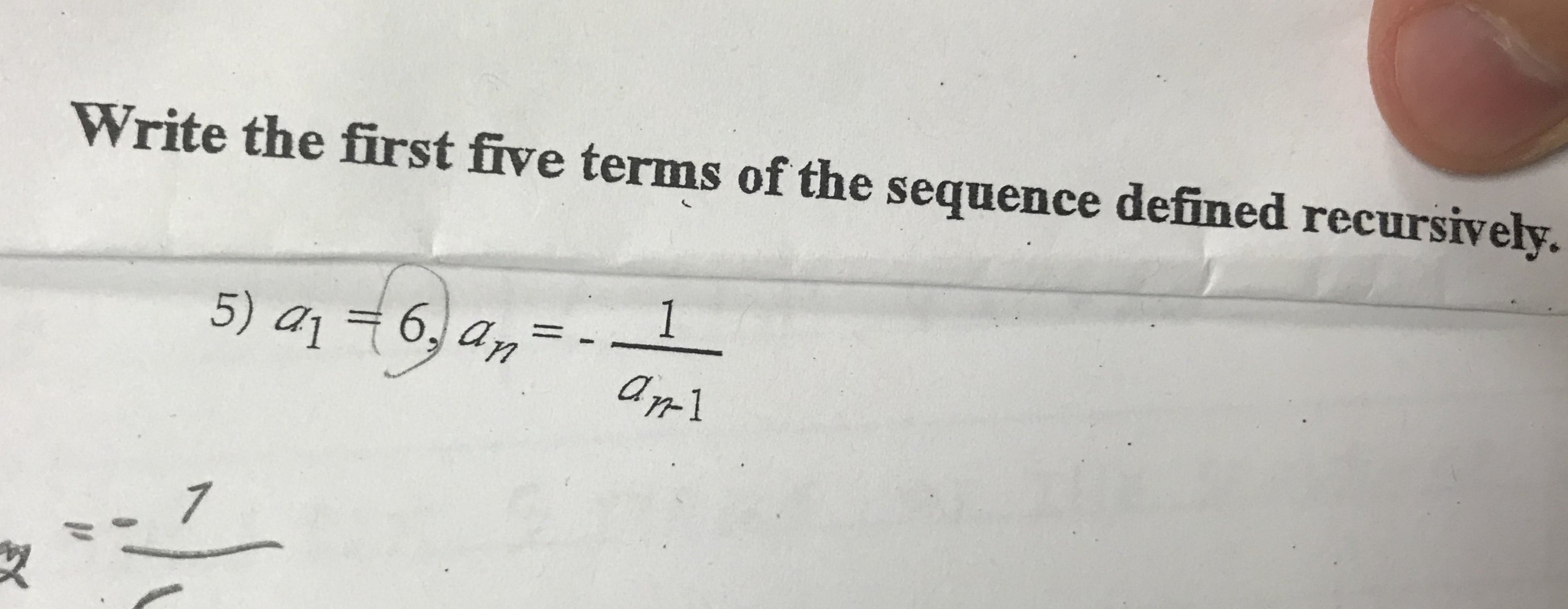# Write the first five terms of the sequence defined recursively.15) 416, amar17

Question
56 viewshelp_outlineImage TranscriptioncloseWrite the first five terms of the sequence defined recursively. 1 5) 416, am ar1 7 fullscreen
check_circle

Step 1

Plug n=2 in the formula to get the second term.

Then plug a1= 6

So, second term = a2= -1/6

Step 2

To get the third term plug n=3.

Then plug a2= -1/6

So, third term = a3= 6

Step 3

Plug n=4 in the formula to get the fourth term.

Then p...

### Want to see the full answer?

See Solution

#### Want to see this answer and more?

Solutions are written by subject experts who are available 24/7. Questions are typically answered within 1 hour.*

See Solution
*Response times may vary by subject and question.
Tagged in

### Other